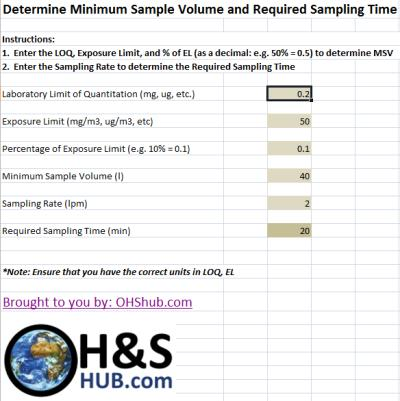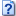# Calculator: Minimum Sample Volume & Required Sampling TimeWant to determine the Minimum Sample Volume needed to ensure you collect enough of a sample volume so that the results are capable of being less than the Exposure Limit? Want to know how long you need to sample at a given flow rate?

If so, use OHShub.com’s Minimum Sample Volume and Required Sampling Time calculator and take the guess-work out of the equation.

Input the:

• Laboratory Limit of Quantitation (LOQ)
• Exposure Limit
• Desired Fraction of the Exposure Limit (essentially a safety factor for sampling)
• Sampling Rate

And the results will give you the Minimum Sample Volume and Required Sampling Time.

Note:  Ensure that you have the right units and all of the units are consistent.  Units such as parts per million (ppm) for Exposure Limits may need to be converted to mg/m3.Calculator: Minimum Sample Volume and Required Sampling Time (35.0 KiB, 3,205 hits)